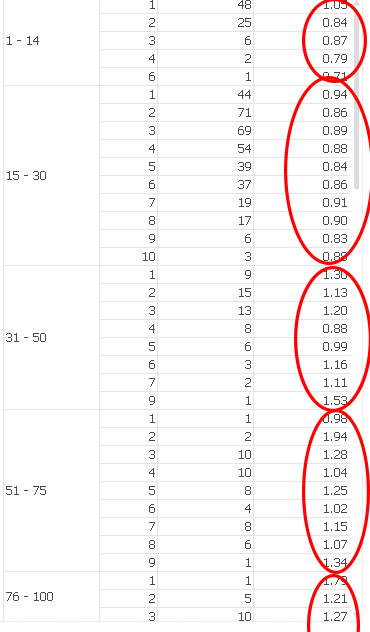# QlikView App Dev

Discussion Board for collaboration related to QlikView App Development.

Announcements
Skip the ticket, Chat with Qlik Support instead for instant assistance.
cancel
Showing results for
Did you mean:Contributor

## Limiting ColorMix

Hey Guys,

I tried to find this but if it exists i must have missed it so sorry if this is a repost.

If i have a pivot table That looks kind of like thisHow do i colormix just the last column so that it is limited to a dimension as indicated below.Basically the default colormix behaviour makes it so that the range is set by the entire dataset. I simply want to highlight the largest number treating each dimension as its own dataset.

Any Idea how i can do this?

Thanks

Simeon

2 RepliesMVP

I am not sure what you mean with the default colormix behaviour, because Colormix1() and Colormix2() functions map to value ranges between 0 and 1 resp. -1 and 1, right?

If you are using the colormix wizard, you need to disable auto range on step 2 and enter expressions for the max and min values per dimension, something like

=Max(Total<YourFirstDimensionField> Value)

=Min(Total<YourFirstDimensionField> Value)Contributor
Author

Right you are i am using the wizard. sorry about calling it default behaviour.

I tried what you suggested but i am still having problems as the expression doesn't work if i do that.

The generated expression looks like this.

ColorMix1 ((1+Sign(2*(rangemin(Max(TOTAL<VenueSize> [To Ratio]),rangemax([To Ratio],min(TOTAL<VenueSize> [To Ratio])))-min(TOTAL<VenueSize> [To Ratio]))/(Max(TOTAL<VenueSize> [To Ratio])-min(TOTAL<VenueSize> [To Ratio]))-1)*Sqrt(Fabs((2*(rangemin(Max(TOTAL<VenueSize> [To Ratio]),rangemax([To Ratio],min(TOTAL<VenueSize> [To Ratio])))-min(TOTAL<VenueSize> [To Ratio]))/(Max(TOTAL<VenueSize> [To Ratio])-min(TOTAL<VenueSize> [To Ratio]))-1))))/2, ARGB(255, 255, 0, 0), ARGB(255, 0, 255, 0))

VenueSize is the first dimension field [To Ratio] is what i am trying to colour mixCommunity Browser